##### SAT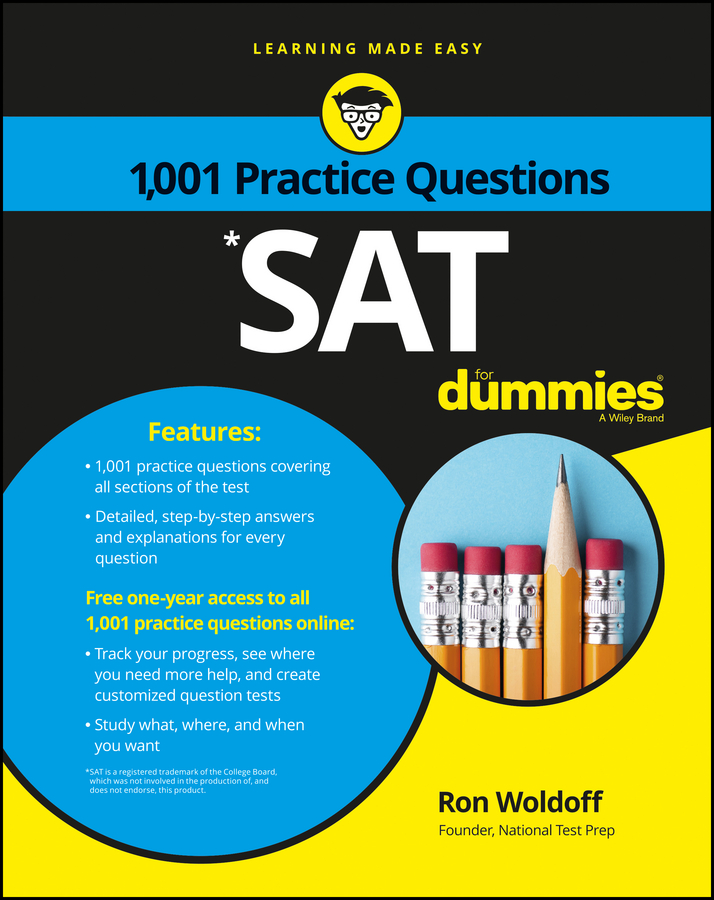Circles are everywhere, especially on the PSAT/NMSQT. It’s no accident that English contains so many references to circles: circle of friends, water circling the drain, coming full circle, and so forth. When you hit a circle question on the PSAT/NMSQT, remember these facts:

• The radius is a straight line from the center of the circle to its edge. In a test question, the radius is represented by the letter r.

• The diameter is a straight line from one edge of the circle to another, passing through the center of the circle. The diameter equals twice the radius.

• The circumference of a circle is the distance around its edge. The formula for circumference is 2πr. The strange symbol is pi and equals approximately 22/7 or approximately 3.14. Generally, when you’re asked to figure out a question with π in it, the answer choices are expressed with π also. You don’t need to multiply by 22/7 or 3.14 or use the π button on your calculator.

Note: This formula appears in the information box on the exam.

• An arc is any portion of the circumference. You may be asked to find the length of an arc. No sweat! First, calculate the entire circumference. Next, figure out the degree-measure of the arc. That’s the angle formed when you draw lines from each end of the arc to the center of the circle.

Usually, the diagram accompanying the question tells you the degree-measure of the arc, as in this figure: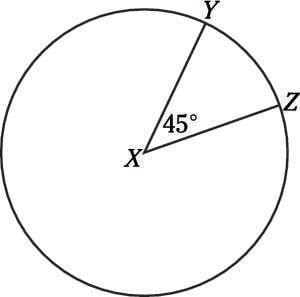The degree-measure of arc YZ is 45°. (If you’re not told the degree-measure, the diagram will contain another clue — a triangle or perhaps a vertical angle that enables you to compute the degree-measure.

Don’t confuse degree-measure with length. You can have a pizza big enough to fill Times Square or one small enough to fit on a child’s plate. A slice of each of those pies may have the same degree-measure, even though you get indigestion after eating the first and hunger pangs after gobbling the second.

Divide the degree-measure by 360. Now you know what fraction of the entire circumference the arc represents. In the figure, divide 45 by 360, which gives you 1/8. Now multiply the circumference by 1/8, and you know how long arc YZ is.

You may not be able to determine the circumference of the circle in normal, how-many-feet-of-carpeting-do-I-need numbers. The circumference you calculate may turn out to be 320π, for example. Then, in the preceding example, the arc measures 1/8 of that number, or 40π.

• The area of a circle is πr2. In other words, square the radius and multiply the result by π. Note: This formula appears in the information box on the exam. You may be asked to find the area of a sector, a wedge cut out of the circle like a piece of pie.

This kind of problem is as easy as, well, pie. Just find the total area of the circle, divide the degree-measure of the sector by 360, and then multiply the total area of the circle by the result. In the preceding figure, for example, imagine that you had enough information to calculate a total area of 800 square feet.

The area of the sector XYZ is 800 x 1/8, or 100 square feet. Or, your answer choice may be 1/8πr2.

Sometimes the PSAT/NMSQT presents you with one shape inside another. Then you have to determine something odd — the area of the shaded portion, perhaps. Don’t panic. The question always contains enough information to give you an answer. First deal with each shape separately and then figure out how the shapes are related.

Workout time! Try these problems:

1. The area of a circle is 50ð. What is the diameter of the circle?

(A) 5
(B)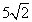(C) 10
(D)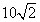(E) 50
2. A wire can be formed into a circle or a square. What is the ratio of the area of the circle to the area of the square?

(A) 1:4
(B) 1:3
(C) 2:π
(D) 4:π
(E) 8:π
3. A 14-inch diameter pizza is cut into slices with 30̊ central angles. Which answer is closest to the combined area of two slices of pizza?

(A) 8 square inches
(B) 13 square inches
(C) 16 square inches
(D) 19 square inches
(E) 26 square inches

1. D.Remember that the area of a circle is πr2, so you can solve for the radius: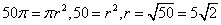Don’t be tricked by Choice (B); remember that you want the diameter, which is twice as long as the radius, or Choice (D).

2. D. 4:ð

This is one of those problems that’s so much easier if you plug in a number; in this case pick a length for the wire — 4, perhaps. If the wire is 4 units long, then the square it forms is 1 unit long on each side and has an area of 1. Bend the 4-unit-long wire into a circle, and the circle’s circumference is 4 units.

Now you can discover the radius using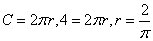The area of that circle is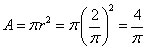The ratio of the area of the circle to the square is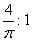But that’s not an option. Multiply both sides by π, and you get Choice (D).

3. E. 26 square inches

The pizza’s total area has to be A = πr2, and the radius is 7 inches (half of the diameter). That means that the area is π(7)2 = 49π = 154 square inches. 30° is 1/12 of 360°, so the area of one slice is 1/12 of 154, or about 12.8 square inches.

You want the area of two slices, so don’t be fooled by Choice (B): 2 x 12.8 is approximately 25.6 inches, or 26 inches, Choice (E).